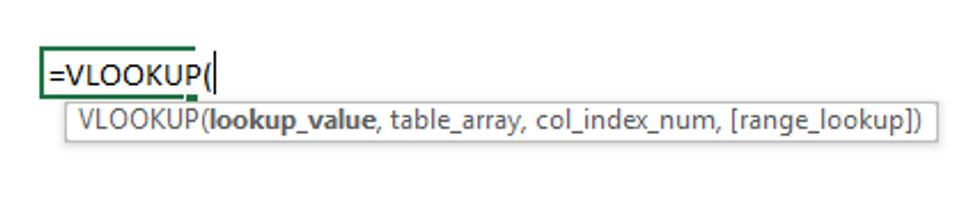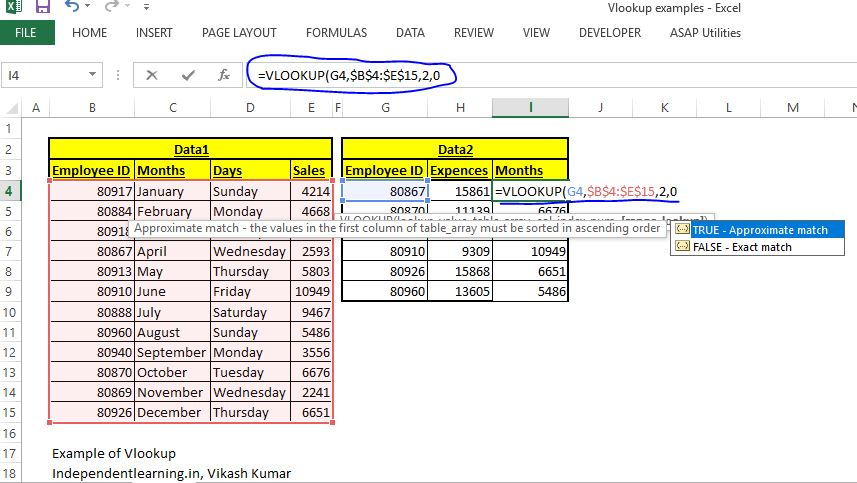## Complete Guide About Vlookup - Syntax, Uses, Limitations and Examples

If you are working on Microsoft excel on huge data in you office, school or collage you must know the USES OF VLOOKUP. When we talk about data lookups, Vlookup is a initial part of the same. Please go through the full article for your better understanding. Must watch the video also.

## Syntax of Vlookup formula

Before Using any excel formula/functions we need to know about the syntax of formula/function. Lets discuss in details syntax of vlookup.Vlookup Syntax

I have attached a image above to describe the syntax of vlookup. Vlookup function have total for objects. Lookup_value, table array, Col_index_num, range_lookup. lets discuss in brief about these.

1. lookup_value - Lookup value the object which use to lookup the data. It is the common values of both the data-sets. This object is the reference.
2. table array -   In function table array is a table from we are going to get the data.
3. col_index_num -  col_index_num is short form of column index number. this object uses for identify the required data column with the reference of lookup_value from the table_array.
4. range_lookup - Range lookup objects uses to get exact match or approximate match of data which we are getting from function. Its always be 0.

## Uses of Vlookup Formula

vlookup excel function is a very useful Microsoft Excel function/formula. If you are working with data you must know about this formula/function. Vlookup basically uses for finding missing details behalf of few given details. in below picture its clearly visible that we have 2 data sets data1 and data2 for sales data for a company xyz inc. In data1 we have four columns (Employee ID, Months, Days, Sales), and second data set we have three columns (Employee ID, Expenses, Sales). But Sales column's data is missing from data2  and we need to fill sales data for given Employee IDs. In this situation we have Employee ID in common so we can use vlookup in this situation.

## Limitations Of Vlookup Function/Formula

Now we know about the uses of vlookup, its time to discuss the limitation of this function. You cannot use vlookup in every case. for the using this function your data must full the requirements for vlookup function. in certain cases you cannot use the vlookup function for lookup requirements. These are few limitations of vlookup formula.
1. Your both data-sets must have a common column. (like Employee id, see the image 1).
2. With v lookup you can only find data Left to right. In simple words if Employee Id column will be moved next to Sales column in data1, vlookup will not work.
3. No duplicates entries in the common column. If there is any duplicate entry will found , result will only for the first entry. (If In Employee Id column first employee id 80917 is twice in the column and it is also in the fifth column (in place of 80913). And when we use vlookup formula will return 4214 in first and fifth column also instead of 5803.

Now we knows about syntax of vlookup, Uses of vlookup and limitations of we lookup. Now we are ready to use this function. Read below for Examples of Vlookup formula

## Examples of Vlookup Function/Formula

In below picture its clearly visible that we have 2 data sets data1 and data2 for sales data for a company xyz inc. In data1 we have four columns (Employee ID, Months, Days, Sales), and second data set we have three columns (Employee ID, Expenses, Sales). But Sales column's data is missing from data2 and we need to fill sales data for given Employee IDs. In this situation we have Employee ID in common so we can use vlookup in this situation.

lets get the data from using vlookup.

I am creating few cases here to understand vlookup. See below the all cases. We will discuss all the case separately in details.

#### Case 1. As mentioned in above image (Vlookup Example) we are going to get sales data.

In this case lookup_value will be Employee ID,
table_array will be whole data1 table,
col_index_number will be 4. (count column of data1 start from Employee id as 1, Months will be 2, Days will be 3 and Sales column number will be 4)

#### Case 2. We are going to get Months column data.

in this case lookup_value will be the same Employee ID,
table_array will be also same, whole data1 table,
col_index_number will be 2 (count column of data1 start from Employee id as 1, Months will be 2, Days will be 3 and Sales column number will be 4)

Then formula will be as shown in below mentioned picture:-Vlookup example
I Hope this Article will help you to understand The Vlookup Formula/Function, its uses. Thanks in advance, i will try to provide few new articles as soon as possible.
Share: